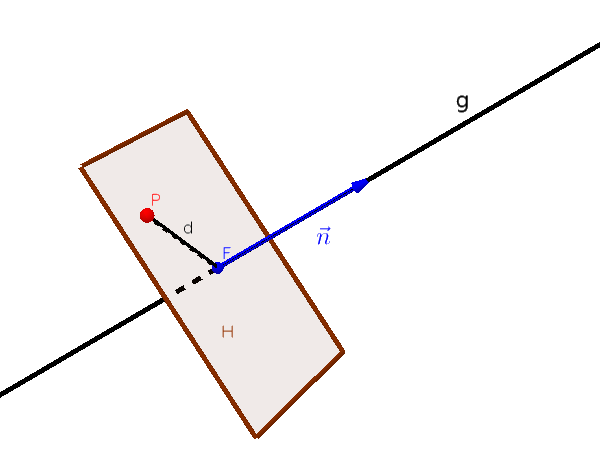Math Distance calculations Point and line

# Distance from point to a line

The distance calculation from a point $P$ to a straight line is somewhat more difficult.Here we need an auxiliary plane $H$ that is orthogonal (perpendicular) to the line $g$ and contains the point $P$.

The intersection point of the line with the auxiliary plane is called the perpendicular point $F$.The distance from perpendicular point $F$ to point $P$ corresponds to the distance from the line to the point and can be easily calculated.

i

### Method

1. Set up the normal equation of the auxiliary plane H (with P and direction vector of g)

2. Calculate perpendicular point F (intersection of line g and plane H)

3. $d$ corresponds to the distance from P to F, ie $|\vec{PF}|$ (length of vector)

### Example

$P(-1|0|3)$

$\text{g: } \vec{x} = \begin{pmatrix} 1 \\ 2 \\ 1 \end{pmatrix} + r \cdot \begin{pmatrix} 1 \\ 1 \\ 0 \end{pmatrix}$

1. #### Set up the auxiliary plane

The auxiliary level should contain point $P$. So that's our support point. We take the direction vector of the straight line as the normal vector $\vec{n}$, since the line and plane should be orthogonal to each other.

$\text{H: } (\vec{x} - \vec{a}) \cdot \vec{n}=0$

$\text{H: } (\vec{x} - \begin{pmatrix} -1 \\ 0 \\ 3 \end{pmatrix}) \cdot \begin{pmatrix} 1 \\ 1 \\ 0 \end{pmatrix}=0$

2. #### Calculate perpendicular point

The perpendicular point is the intersection of the line $g$ and the auxiliary plane $H$. To calculate the intersection, the equation of a line for $\vec{x}$ is used in the plane.

$\left(\color{red}{\begin{pmatrix} 1 \\ 2 \\ 1 \end{pmatrix} + r \cdot \begin{pmatrix} 1 \\ 1 \\ 0 \end{pmatrix}} - \begin{pmatrix} -1 \\ 0 \\ 3 \end{pmatrix}\right)$ $\cdot \begin{pmatrix} 1 \\ 1 \\ 0 \end{pmatrix}=0$

$\begin{pmatrix} 2+r \\ 2+r \\ -2 \end{pmatrix}$ $\cdot \begin{pmatrix} 1 \\ 1 \\ 0 \end{pmatrix}=0$

Calculate scalar product

$(2+r)\cdot1+(2+r)\cdot1$ $+(-2)\cdot0=0$
$4+2r=0\quad|-4$
$2r=-4\quad|:2$
$r=-2$

Insert $r$ in $g$ to get perpendicular point $F$

$\vec{OF} = \begin{pmatrix} 1 \\ 2 \\ 1 \end{pmatrix} \color{red}{-2} \cdot \begin{pmatrix} 1 \\ 1 \\ 0 \end{pmatrix}$ $= \begin{pmatrix} 1-2 \\ 2-2 \\ 1-0 \end{pmatrix}$ $= \begin{pmatrix} -1 \\ 0 \\ 1 \end{pmatrix}$

$F(-1|0|1)$

3. #### Calculate the distance between the two points

The distance from the perpendicular point to point $P$ is also the distance from the line to this point.

The distance between two points can be easily calculated using vectors.

$d=|\vec{PF}|$ $=\left| \begin{pmatrix} -1-(-1) \\ 0-0 \\ 1-3 \end{pmatrix}\right|$ $=\left| \begin{pmatrix} 0 \\ 0 \\ -2 \end{pmatrix}\right|$ $=\sqrt{(-2)^2}$ $=2$

The distance from the line $g$ to the point $P$ is 2 LU.